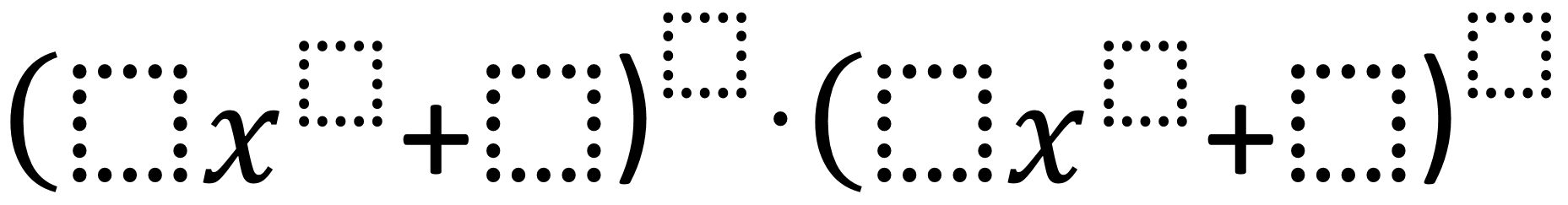# Highest Degree Polynomials

Directions: Using the digits 1 to 9 at most one time each, fill in the boxes to make a polynomial of the highest degree.### Hint

Where should you put the 9?

### Answer

One binomial must have an 8 and 9 for the two exponents and the other must have 7 and 6.  The values of the coefficient and constants are irrelevant.

Source: Robert Kaplinsky

## Writing Equivalent Polynomial Expressions

Directions: Use the digits 1-9, at most one time each, to create a true statement. …